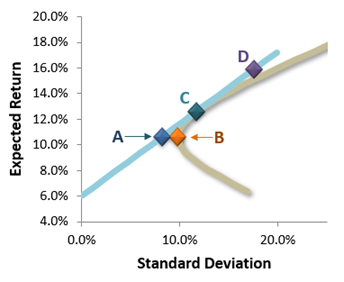# P2.T9.21.2. Ang's volatility and risk anomalies

#### Nicole Seaman

##### Director of FRM Operations
Staff member
Subscriber
Learning objectives: Describe issues that arise when measuring alphas for nonlinear strategies. Compare the volatility anomaly and the beta anomaly and analyze evidence of each anomaly. Describe potential explanations for the risk anomaly.

Questions:

21.2.1. Robert runs a portfolio with a focused strategy of selling put options. To evaluate his portfolio, he runs a CAPM regression of the typical sort: the portfolio's excess returns regressed against the market's excess returns. Because the portfolio has a four-year track record, his regression is based on returns over the most recent 48 months. His regression result is given by R(p) = +0.0520 + 0.67 × R(m) + ε. Based on the result, he declares that his portfolio generates an alpha of +5.20%. His colleague Mary criticizes this conclusion. Which of the following is probably the BEST criticism of Robert's approach?

a. It is impossible to evaluate nonlinear payoffs
b. Alpha is the residual term, not the regression intercept
c. The strategy's return distribution is very negatively skewed
d. Returns are distorted by the short put strategy that collects up-front cash premiums

21.2.2. Betty is the Chief Investment Officer (CIO) at a fund of funds manager. She is evaluating whether to invest in Acme Fund's highly sophisticated active strategy. This strategy is highly discretionary and allows for exposure to multiple factors, including but not limited to value, size, momentum, and volatility, among several others. Her staff analyst John runs almost 100 regressions (of this active strategy) against different sets of factors; i.e., regressions of the strategy's five-year performance against the factor benchmark. He discovers a set of factors that demonstrates Acme's strategy generates zero alpha. According to Ang, what is the key problem with John's analysis?

a. There is no theoretical problem
b. Alpha and the benchmark are simultaneously determined
c. It is typically impossible to find an ex-post benchmark portfolio the produces no alpha
d. Grossman and Stiglitz demonstrated that markets are perfectly efficient such that alpha does not exist

21.2.3. Derek has constructed a portfolio possibilities curve (PPV) which is plotted below. The risk-free rate assumption is 6.0%.• Portfolio (A) has the lowest volatility (among the four portfolios) but it has a low realized beta
• Portfolio (B) has the second-lowest volatility but it has a high realized beta
• Portfolio (C) has the highest Sharpe ratio among portfolios on the PPC
• Portfolio (D) borrows to add leverage to Portfolio (C)
If we assume the validity of the low-risk anomaly, what portfolio is likely to offer the best returns?

a. Portfolio A
b. Portfolio B
c. Portfolio C
d. Portfolio D